Next: Calculation of the Output Up: A Modular Neural Previous: The Network Architecture

Training the System

The training occurs in two stages. All the modules are trained using the Backpropagation algorithm .

In the first phase all sub-networks in the input layer are trained. The training set for each sub-network is selected from the original training set. The training pair for a single module consists of the components of the original vector which are connected to this particular network (as input vector) together with the desired output class represented in binary coding.

All input modules can be trained in parallel very easily because they are all mutually independent.

In the second stage the decision network is trained. The training set for the decision module is built from the output of the input layer together with the original class number. To calculate the set each original input pattern is applied to the input layer; the resulting vector together with the desired output class (represented in a 1-out-of-k coding) form the training pair for the decision module.

The original training set is:for all. Where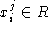is the ith component of the jth input vector,is the class number, and t is the number of training instances.

The module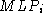is connected to::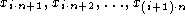The training set for the network: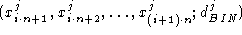for allThe mapping performed by the input layer: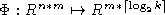.

The training set for the decision network: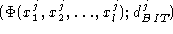and.

The mapping of the decision network: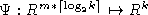.

Albrecht Schmidt and Zuhair Bandar
Wed Apr 16 13:57:11 MET DST 1997